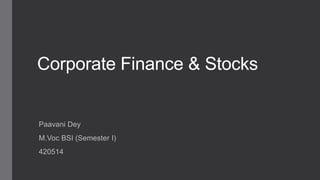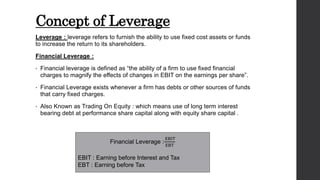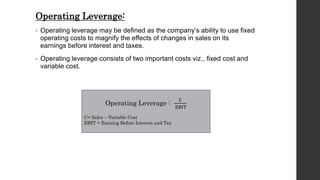Seu SlideShare está sendo baixado. ×

# Leverage and Capital Structure .pptx

Anúncio
Anúncio
Anúncio
Anúncio
Anúncio
Anúncio
Anúncio
Anúncio
Anúncio
Anúncio
AnúncioCarregando em…3
×

1 de 16 Anúncio

# Leverage and Capital Structure .pptx

Well arranged presentation of Concept of Leverage and Concept of Capital Structure and its Theories.

Well arranged presentation of Concept of Leverage and Concept of Capital Structure and its Theories.

Anúncio
Anúncio

### Leverage and Capital Structure .pptx

1. 1. Corporate Finance & Stocks Paavani Dey M.Voc BSI (Semester I) 420514
2. 2. Concept of Leverage Leverage : leverage refers to furnish the ability to use fixed cost assets or funds to increase the return to its shareholders. Financial Leverage : • Financial leverage is defined as “the ability of a firm to use fixed financial charges to magnify the effects of changes in EBIT on the earnings per share”. • Financial Leverage exists whenever a firm has debts or other sources of funds that carry fixed charges. • Also Known as Trading On Equity : which means use of long term interest bearing debt at performance share capital along with equity share capital . Financial Leverage : EBIT EBT EBIT : Earning before Interest and Tax EBT : Earning before Tax
3. 3. Operating Leverage: • Operating leverage may be defined as the company’s ability to use fixed operating costs to magnify the effects of changes in sales on its earnings before interest and taxes. • Operating leverage consists of two important costs viz., fixed cost and variable cost. Operating Leverage : C EBIT C= Sales – Variable Cost EBIT = Earning Before Interest and Tax
4. 4. COMBINED LEVERAGE : • When the company uses both financial and operating leverage to magnification of any change in sales into a larger relative changes in earning per share. Combined leverage is also called as composite leverage or total leverage. • It clarify about the combined effect of operating and financial leverage. • Combined leverage can be calculated with the help of the following formulas: CL = OL × FL OL = Operating Leverage FL= Financial Leverage
5. 5. Capital Structure • Capital Structure refer to the proportion between the various long term sources of finance in the total capital of firm . • A financial manager choose that source of finance which include minimum risk as well as minimum cost of capital. • Objectives of Capital Structure Decision of capital structure aims at the following two important objectives: I. Minimum the cost of capital II. Maximum the value of the firm. • Optimum capital structure may be defined as the capital structure or combination of debt and equity, that leads to the maximum value of the firm.
6. 6. FACTORS DETERMINING CAPITAL STRUCTURE The following factors are considered while deciding the capital structure of the firm. • Leverage :It is the basic and important factor, which affect the capital structure. It uses the fixed cost financing such as debt, equity and preference share capital. It is closely related to the overall cost of capital. • Cost of Capital :Cost of capital constitutes the major part for deciding the capital structure of a firm. Normally long- term finance such as equity and debt consist of fixed cost while mobilization. When the cost of capital increases, value of the firm will also decrease. Hence the firm must take careful steps to reduce the cost of capital. • Nature of the business: Use of fixed interest/dividend bearing finance depends upon the nature of the business. If the business consists of long period of operation, it will apply for equity than debt, and it will reduce the cost of capital. • Size of the company: It also affects the capital structure of a firm. If the firm belongs to large scale, it can manage the financial requirements with the help of internal sources. But if it is small size, they will go for external finance. It consists of high cost of capital. • Legal requirements: Legal requirements are also one of the considerations while dividing the capital structure of a firm. For example, banking companies are restricted to raise funds from some sources. • Requirement of investors: In order to collect funds from different type of investors, it will be appropriate for the companies to issue different sources of securities. • Government policy : Promoter contribution is fixed by the company Act. It restricts to mobilize large, long term funds from external sources. Hence the company must consider government policy regarding the capital structure.
7. 7. Capital Structure Theories • Net Income Approach • Traditional Approach • Modigliani Miller Approach
8. 8. Net Income Approach • Net income approach suggested by the Durand. • According to this approach, the capital structure decision is relevant to the valuation of the firm. • The increase in the proportion of debt capital in the capital structure brings a decrease in overall cost of capital because debt is a cheaper Source of finance. • The decrease in cost of capital, in turn, increases the value of the firm. • Thus, when the proportion of debt is 100% in capital structure, cost of capital is minimum. And when there is no debt in capital structure, the cost of capital is maximum. • Net income approach is based on the following three important assumptions: 1. There are no corporate taxes. 2. The cost debt is less than the cost of equity. 3. The use of debt does not change the risk perception of the investor. 4. There are only two sources of finance i.e. debt and equity.
9. 9. Contd. Formula : V = S+D • V = Value of firm • S = Market value of equity • D = Market value of debt Market value of the equity can be ascertained by the following formula: S = NI 𝐾 where • NI = Earnings available to equity shareholder • K = Cost of equity/equity capitalization rate
10. 10. Traditional Approach • It is the mix of Net Income approach and Net Operating Income approach. • Hence, it is also called as intermediate approach. • According to the traditional approach, mix of debt and equity capital can increase the value of the firm by reducing overall cost of capital up to certain level of debt. • Traditional approach states that the overall cost of capital decreases only within the responsible limit of financial leverage and when reaching the minimum level, it starts increasing with financial leverage. • Capital structure theories are based on certain assumption :  There are only two sources of funds used by a firm; debt and shares.  The firm pays 100% of its earning as dividend.  The total assets are given and do not change.  The total finance remains constant.  The operating profits (EBIT) are not expected to grow.  The business risk remains constant.  The firm has a perpetual life.  The investors behave rationally.
11. 11. Contd. Three stage of capital structure under traditional approach • Stage 1: The value of the firm may first increase with moderate leverage when WACC is decreasing. • Stage 2: Reach the maximum value when WACC is minimum. • Stage 3: Then starts declining with higher financial leverage when WACC start increasing.
12. 12. Contd. Formulas Value Of The Firm(V) = Db + Eq • Db=market value of debt • Eq= market value of equity Market Value Of Equity (Eq) = EBT/ Ke • EBT = EBIT - Interest • Ke = Cost of equity
13. 13. • According to MM Approach cost of capital is independent of capital structure and financial leverage does not affect the overall cost of capital and hence there is no optimum capital structure. • Modigliani and Miller approach states that the financing decision of a firm does not affect the market value of a firm in a perfect capital market. • In other words MM approach maintains that the average cost of capital does not change with change in the debt weighted equity mix or capital structures of the firm. • Modigliani and Miller approach is based on the following important assumptions:  There is a perfect capital market.  There are no retained earnings.  There are no corporate taxes.  The investors act rationally.  The dividend payout ratio is 100%.  The business consists of the same level of business risk. Modigliani Miller Approach
14. 14. Contd. MM Model proposition : • Value of a firm is independent of the capital structure. • Value of firm is equal to the capitalized value of operating income (i.e. EBIT) by the appropriate rate (i.e. WACC). • Value of Firm = Mkt. Value of Equity + Mkt. Value of Debt = Expected EBIT / Expected WACC • As per MM, identical firms (except capital structure) will have the same level of earnings. • If market values of identical firms are different, ‘arbitrage process’ will take place the difference will mitigate. • In the ‘arbitrage process’ investors will switch their securities between identical firms (from levered firms to un-levered firms) and receive the same returns from both firms.
15. 15. Contd. Formulas Value Of The Firm(V) = Db + Eq • Db = Mkt value of debt • Eq = Mkt value of Equity Market Value Of Equity (Eq) = EBT / Ke • EBT = EBIT - Interest • Ke = Cost of equity
16. 16. Thank you .